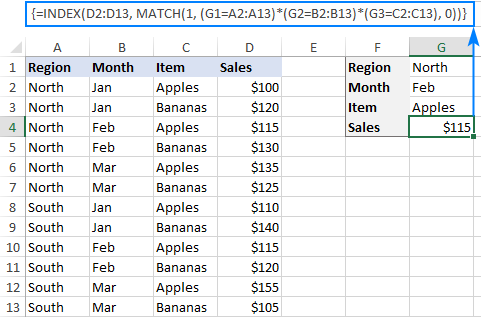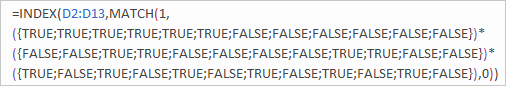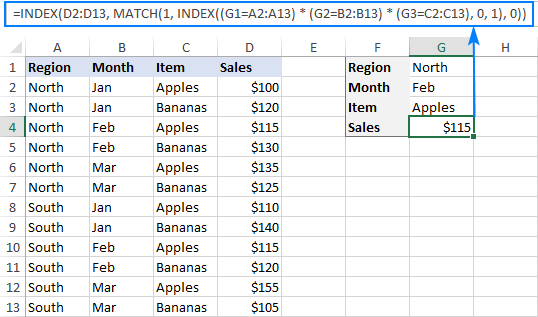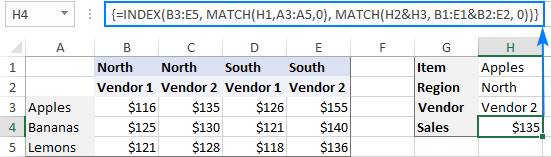# Excel INDEX MATCH with multiple criteria - formula examples

The tutorial shows how to lookup with multiple criteria in Excel using INDEX and MATCH and a few other ways.

Although Microsoft Excel provides special functions for vertical and horizontal lookup, expert users normally replace them with INDEX MATCH, which is superior to VLOOKUP and HLOOKUP in many ways. Among other things, it can look up two or more criteria in columns and rows. This tutorial explains the syntax and inner mechanics in full detail so that you can easily adjust the formula for your particular needs. To make the examples easier to follow, you are welcome to download our sample workbook.

## Excel INDEX MATCH with multiple criteria

When working with large databases, you may sometimes find yourself in a situation when you need to find something but don't have a unique identifier for the search. In this case, lookup with several conditions is the only solution.

To look up a value based on multiple criteria in separate columns, use this generic formula:

{=INDEX(return_range, MATCH(1, (criteria1=range1) * (criteria2=range2) * (…), 0))}

Where:

• Return_range is the range from which to return a value.
• Criteria1, criteria2, … are the conditions to be met.
• Range1, range2, … are the ranges on which the corresponding criteria should be tested.
Important note! This is an array formula and it must be completed with Ctrl + Shift + Enter. This will enclose your formula in {curly brackets}, which is a visual sign of an array formula in Excel. Do not try typing the braces manually, that won't work!

The formula is an advanced version of the iconic INDEX MATCH that returns a match based on a single criterion. To evaluate multiple criteria, we use the multiplication operation that works as the AND operator in array formulas. Below, you will find a real-life example and the detailed explanation of the logic.

### INDEX MATCH with several criteria - formula example

For this example, we will be using a table in the so-called "flat-file" format with each separate criteria combination (region-month-item in our case) on its own row. Our goal is to retrieve the sales figure for a certain item in a specific region and month.

With the source data and criteria in the following cells:

• Return_range (sales) - D2:D13
• Criteria1 (target region) - G1
• Criteria2 (target month) - G2
• Criteria3 (target item) - G3
• Range1 (regions) - A2:A13
• Range2 (months) - B2:B13
• Range3 (items) - C2:C13

The formula takes the following shape:

`=INDEX(D2:D13, MATCH(1, (G1=A2:A13) * (G2=B2:B13) * (G3=C2:C13), 0))`

Enter the formula, say in G4, complete it by pressing Ctrl + Shift + Enter and you will get the following result:### How this formula works

The trickiest part is the MATCH function, so let's figure it out first:

`MATCH(1, (G1=A2:A13) * (G2=B2:B13) * (G3=C2:C13), 0))`

As you may remember, MATCH(lookup_value, lookup_array, [match_type]) searches for the lookup value in the lookup array and returns the relative position of that value in the array.

In our formula, the arguments are as follows:

• Lookup_value: 1
• Lookup_array: (G1=A2:A13) * (G2=B2:B13) * (G3=C2:C13)
• Match_type: 0

The 1st argument is crystal clear - the function searches for the number 1.  The 3rd argument set to 0 means an "exact match", i.e. the formula returns the first found value that is exactly equal to the lookup value.

The question is - why do we search for "1"? To get the answer, let's have a closer look at the lookup array where we compare each criterion against the corresponding range: the target region in G1 against all regions (A2:A13), the target month in G2 against all months (B2:B13) and the target item in G3 against all items (C2:C13).  An intermediate result is 3 arrays of TRUE and FALSE where TRUE represents values that meet the tested condition. To visualize this, you can select the individual expressions in the formula and press the F9 key to see what each expression evaluates to:The multiplication operation transforms the TRUE and FALSE values into 1's and 0's, respectively:

`{1;1;1;1;1;1;0;0;0;0;0;0} * {0;0;1;1;0;0;0;0;1;1;0;0} * {1;0;1;0;1;0;1;0;1;0;1;0}`

And because multiplying by 0 always gives 0, the resulting array has 1's only in the rows that meet all the criteria:

`{0;0;1;0;0;0;0;0;0;0;0;0}`

The above array goes to the lookup_array argument of MATCH. With lookup_value of 1, the function returns the relative position of the row for which all the criteria are TRUE (row 3 in our case). If there are several 1's in the array, the position of the first one is returned.

The number returned by MATCH goes directly to the row_num argument of the INDEX(array, row_num, [column_num]) function:

`=INDEX(D2:D13, 3)`

And it yields a result of \$115, which is the 3rd value in the D2:D13 array.

## Non-array INDEX MATCH formula with multiple criteria

The array formula discussed in the previous example works nice for experienced users. But if you are building a formula for someone else and that someone does not know array functions, they may inadvertently break it. For example, a user may click your formula to examine it, and then press Enter instead of Ctrl + Shift + Enter. In such cases, it would be wise to avoid arrays and use a regular formula that is more bulletproof:

INDEX(return_range, MATCH(1, INDEX((criteria1=range1) * (criteria2=range2) * (..), 0, 1), 0))

For our sample dataset, the formula goes as follows:

`=INDEX(D2:D13, MATCH(1, INDEX((G1=A2:A13) * (G2=B2:B13) * (G3=C2:C13), 0, 1), 0))`### How this formula works

As the INDEX function can process arrays natively, we add another INDEX to handle the array of 1's and 0's that is created by multiplying two or more TRUE/FALSE arrays. The second INDEX is configured with 0 row_num argument for the formula to return the entire column array rather than a single value. Since it's a one-column array anyway, we can safely supply 1 for column_num:

`INDEX({0;0;1;0;0;0;0;0;0;0;0;0}, 0, 1) returns {0;0;1;0;0;0;0;0;0;0;0;0}`

This array is passed to the MATCH function:

`MATCH(1, {0;0;1;0;0;0;0;0;0;0;0;0}, 0)`

MATCH finds the row number for which all the criteria are TRUE (more precisely, the the relative position of that row in the specified array) and passes that number to the row_num argument of the first INDEX:

`=INDEX(D2:D13, 3)`

## INDEX MATCH with multiple criteria in rows and columns

This example shows how to perform lookup by testing two or more criteria in rows and columns. In fact, it's a more complex case of the so-called "matrix lookup" or "two-way lookup" with more than one header row.

Here's the generic INDEX MATCH formula with multiple criteria in rows and columns:

{=INDEX(table_array, MATCH(vlookup_value, lookup_column, 0), MATCH(hlookup_value1 & hlookup_value2, lookup_row1 & lookup_row2, 0))}

Where:

Table_array - the map or area to search within, i.e. all data values excluding column and rows headers.

Vlookup_value - the value you are looking for vertically in a column.

Lookup_column - the column range to search in, usually the row headers.

Hlookup_value1, hlookup_value2, …  - the values you are looking for horizontally in rows.

Lookup_row1, lookup_row2, … - the row ranges to search in, usually the column headers.

Important note! For the formula to work correctly, it must be entered as an array formula with Ctrl + Shift + Enter.

It is a variation of the classic two-way lookup formula that searches for a value at the intersection of a certain row and column. The difference is that you concatenate several hlookup values and ranges to evaluate multiple column headers. To better understand the logic, please consider the following example.

### Matrix lookup with multiple criteria - formula example

In the sample table below, we'll be searching for a value based on the row headers (Items) and 2 column headers (Regions and Vendors). To make the formula easier to build, let's first define all the criteria and ranges:

• Table_array - B3:E4
• Vlookup_value (target item) - H1
• Lookup_column (Row headers: items) - A3:A4
• Hlookup_value1 (target region) - H2
• Hlookup_value2 (target vendor) - H3
• Lookup_row1 (Column headers 1: regions) - B1:E1
• Lookup_row2 (Column headers 2: vendors) - B2:E2

And now, supply the arguments into the generic formula explained above, and you will get this result:

`=INDEX(B3:E5, MATCH(H1,A3:A5,0), MATCH(H2&H3,B1:E1&B2:E2,0))`

Remember to complete the formula by pressing the Ctrl + Shift + Enter shortcut, and your matrix lookup with multiple criteria will be done successfully:### How this formula works

As we are searching vertically and horizontally, we need to supply both the row and column numbers for the INDEX(array, row_num, column_num) function.

Row_num is delivered by MATCH(H1, A3:A5, 0) that compares the target item (Apples) in H1 against the row headers in A3:A5. This gives a result of 1 because "Apples" is the 1st item in the specified range.

Column_num is worked out by concatenating 2 lookup values and 2 lookup arrays: MATCH(H2&H3, B1:E1&B2:E2, 0))

The key factor for success is that the lookup values should match the column headers exactly and be concatenated in the same order. To visualize this, select the first two arguments in the MATCH formula, press F9, and you will see what each argument evaluates to:

`MATCH("NorthVendor 2", {"NorthVendor 1", "NorthVendor 2", "SouthVendor 1", "SouthVendor 2"}, 0)`

As "NorthVendor 2" is the second element in the array, the function returns 2.

At this point, our lengthy two-dimensional INDEX MATCH formula transforms into this simple one:

`=INDEX(B3:E5, 1, 2)`

And returns a value at the intersection of the 1st row and 2nd column in the range B3:E5, which is the value in the cell C3.

That's how to look up multiple criteria in Excel. I thank you for reading and hope to see you on our blog next week!

Please feel free to download Excel INDEX MATCH multiple criteria sample workbook with all the formulas discussed in this tutorial.

## Other ways to look up with several conditions in Excel

VLOOKUP with multiple criteria – this requires adding a helper column to the left of your table and concatenating all criteria in that column. That's not a very elegant solution, but you may want to know all available options.

XLOOKUP with multiple criteria – this recent addition to the family of Excel's lookup and reference functions (currently available only to Office 365 subscribers) handles arrays by design. Meaning, it works as a regular formula, not an array formula!

Lookup multiple criteria and return multiple results - combine 5 different functions in a single formula to evaluate several criteria and return all matches either in a column or row.

Merge Tables Wizard - visual way to Vlookup in Excel with one or more criteria.

### 2 Responses to "Excel INDEX MATCH with multiple criteria - formula examples"

1.kanth reddy says:

why should not try like this type
INDEX(E3:E14,MATCH(TRUE,COUNTIFS(B3:B14,H2,C3:C14,H3,D3:D14,H4),0))
INDEX(E3:E14,MATCH(1,(--(COUNTIFS(B3:B14,H2,C3:C14,H3,D3:D14,H4))),0))

•Svetlana Cheusheva (Ablebits.com Team) says:

Hi Kanth,